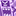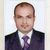# QlikView App Dev

Discussion Board for collaboration related to QlikView App Development.

Announcements
Coming Aug. 9: New Simplified Authoring for Qlik Sense SaaS – For Details, CLICK HERE
cancel
Showing results for
Did you mean:Contributor III

## Set analysis - division

Hi,

If I have to calculate sum(x)/sum(y) for the latest month in a text box would I have to do it this way

sum({<[Month ] = {'\$(VMaxMonth)'}>}x) /sum({<[Month ] = {'\$(VMaxMonth)'}>}y)

1 Solution

Accepted SolutionsMVP

Normally, This?

Sum({<Month = {'\$(=Max(Month))'}>} (x/y))

With Variable, This?

sum({<Month = {'\$(VMaxMonth)'}>} (x/y))

Before develop something, think If placed (The Right information | To the right people | At the Right time | In the Right place | With the Right context)
13 RepliesMVP

what is the Format of your month?MVP

Normally, This?

Sum({<Month = {'\$(=Max(Month))'}>} (x/y))

With Variable, This?

sum({<Month = {'\$(VMaxMonth)'}>} (x/y))

Before develop something, think If placed (The Right information | To the right people | At the Right time | In the Right place | With the Right context)Specialist II

you can also use FirstSortedValue function. A sample data would be helpful hereMVP

Did you have num month field if not please introduce in data model

*,

Num(Month(DateField)) as NumMonth

From Source;

On the UI try this

sum({<NumMonth = { "\$(=Max(NumMonth)" }>}x)  / sum({<NumMonth = { "\$(=Max(NumMonth))" }>}y)Contributor III
Author

How do we use this function in this case

consider the following data

1            100                       90

2             200                     100

I want to get the month 2 data in this format 100/200.MVP

Another way try this also for latest month

sum({<NumMonth = { "\$(=Num(Month(Today()))" }>}x)  / sum({<NumMonth = { "\$(=Num(Month(Today())))" }>}y)MVP

try this

sum({<[Month ] = {"\$(=max(Month))"}>}x) /sum({<[Month ] = {"\$(=max(Month))"}>}y)MVP

Then try this if Month is NUM field

sum({<[Month ] = {"\$(=max(Month))"}>} ReceivedFIles) / sum({<[Month ] = {"\$(=max(Month))"} >} SentFiles)Specialist II